### Home > INT3 > Chapter 7 > Lesson 7.1.4 > Problem7-43

7-43.
1. A rule-of-thumb used by car dealers is that the trade-in value of a car decreases by 20% each year. Homework Help ✎

1. Explain how the phrase “decreases by 20% each year” tells you that the trade-in value decreases exponentially with time (i.e., can be represented by an exponential function).

2.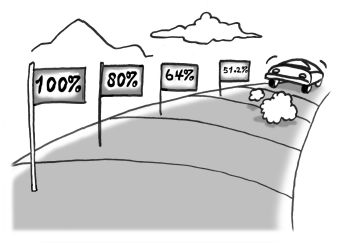Suppose the initial value of your car is $23,500. Write an equation expressing the trade-in value of your car as a function of the number of years from now. 3. How much will your car be worth in four years? 4. What is the average rate of change in the value of your car during the first four years? Explain what the average rate of change means in this context. 5. In how many years will the trade-in value of your car be$6000?

6. If your car is really 2.7 years old now, what was its value when it was new?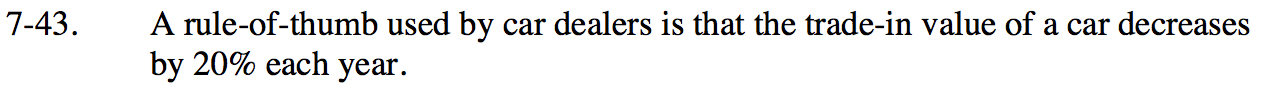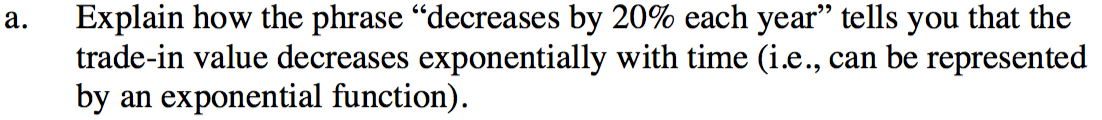If you start with 100% and decrease by 20%, what percentage remains? This is the multiplier.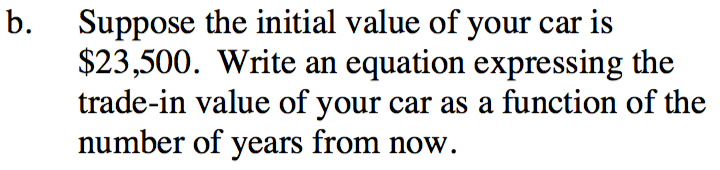y = 23500(0.8)x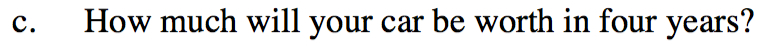Let x = 4 in the equation from part (b).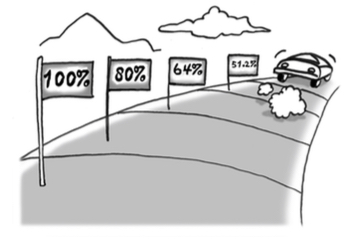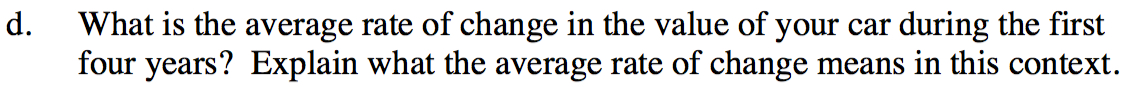What was the total change in value during the first four years? Divide this number by 4.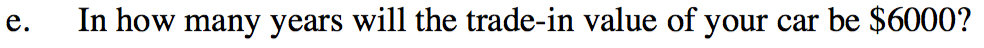Solve: 6000 = 23500(0.8)x

Divide by 23,500 first then take the log of both sides.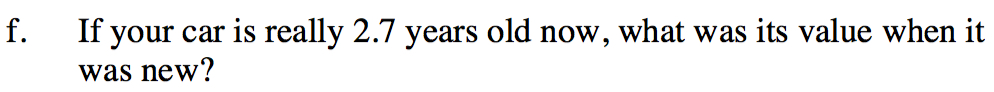This was 2.7 years ago, so let x = −2.7 in the equation.